College Physics : Capacitors

Example Questions

Example Question #1 : Circuits

Which of the following expressions gives the capacitance for a capacitor in a circuit in which the only factors known are a) the current through the circuit, b) the resistance of the circuit, and c) the charge that has accumulated on the capacitor?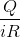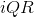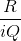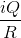Explanation:

In this question, we're given a number of parameters of a circuit and are asked to find how we can use these various parameters to show the capacitance of the circuit's capacitor.

First, recall what a capacitor is; something that stores charge for a given voltage difference. In other words, when there is a voltage difference between the two plates of a capacitor, a certain amount of charge can be stored on these plates. The more charge that can be stored for a given voltage difference, the greater that capacitor's capacitance. This can be shown by the following equation.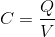From the above expression,is the capacitance which we are trying to find a proper expression for.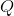is the charge accumulated on the plates, which is one parameter we're given. Voltage,, on the other hand, is not provided.

To put the voltage into different terms, we'll need to use Ohm's law, which states the following.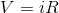In other words, the voltage is proportional to both the current and the resistance of the circuit.

Since we are given both current and resistance as known parameters in the question stem, we can use these for our final answer. By substituting thein the capacitance expression with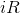, we obtain the following answer.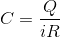Example Question #11 : Electromagnetics

A parallel plate capacitor with no dielectric has capacitance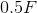. The distance between the capacitor plates is halved. What is the new capacitance?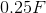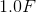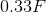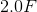Explanation:

The capacitance of a parallel plate capacitor is proportional to the inverse of the distance between the plates. If the distance is halved, the capacitance is doubled.

Example Question #95 : College Physics

An RC circuit is connected to a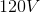power supply. If the resistance of the resistor is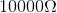and the capacitance is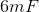, how long will it take for this capacitor to become fully charged?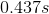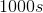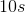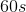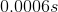Explanation:

The formula for finding the voltage of a simple RC circuit is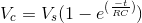, where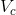is the capacitor voltage,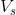is the source voltage,is the resistance, andis the capacitance.

We want to know when the capacitor will reach the voltage of the power source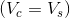so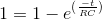and thus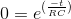Using the properties of natural logs yields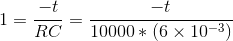Solving foryields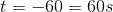All College Physics Resources